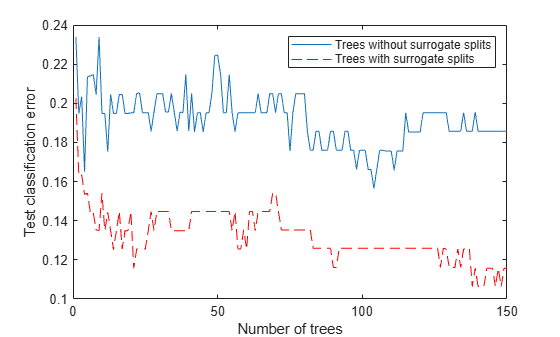Surrogate Splits

When the value of the optimal split predictor for an observation is missing, if you specify to use surrogate splits, the software sends the observation to the left or right child node using the best surrogate predictor. When you have missing data, trees and ensembles of trees with surrogate splits give better predictions. This example shows how to improve the accuracy of predictions for data with missing values by using decision trees with surrogate splits.

Partition the data set into training and test sets. Hold out 30% of the data for testing.

rng('default') % For reproducibility
cv = cvpartition(Y,'Holdout',0.3);

Identify the training and testing data.

Xtrain = X(training(cv),:);
Ytrain = Y(training(cv));
Xtest = X(test(cv),:);
Ytest = Y(test(cv));

Suppose half of the values in the test set are missing. Set half of the values in the test set to NaN.

Xtest(rand(size(Xtest))>0.5) = NaN;

Train Random Forest

Train a random forest of 150 classification trees without surrogate splits.

templ = templateTree('Reproducible',true);  % For reproducibility of random predictor selections
Mdl = fitcensemble(Xtrain,Ytrain,'Method','Bag','NumLearningCycles',150,'Learners',templ);

Create a decision tree template that uses surrogate splits. A tree using surrogate splits does not discard the entire observation when it includes missing data in some predictors.

templS = templateTree('Surrogate','On','Reproducible',true);

Train a random forest using the template templS.

Mdls = fitcensemble(Xtrain,Ytrain,'Method','Bag','NumLearningCycles',150,'Learners',templS);

Test Accuracy

Test the accuracy of predictions with and without surrogate splits.

Predict responses and create confusion matrix charts using both approaches.

Ytest_pred = predict(Mdl,Xtest);
figure
cm = confusionchart(Ytest,Ytest_pred);
cm.Title = 'Model Without Surrogates';Ytest_preds = predict(Mdls,Xtest);
figure
cms = confusionchart(Ytest,Ytest_preds);
cms.Title = 'Model with Surrogates';All off-diagonal elements on the confusion matrix represent misclassified data. A good classifier yields a confusion matrix that looks dominantly diagonal. In this case, the classification error is lower for the model trained with surrogate splits.

Estimate cumulative classification errors. Specify 'Mode','Cumulative' when estimating classification errors by using the loss function. The loss function returns a vector in which element J indicates the error using the first J learners.

figure
plot(loss(Mdl,Xtest,Ytest,'Mode','Cumulative'))
hold on
plot(loss(Mdls,Xtest,Ytest,'Mode','Cumulative'),'r--')
legend('Trees without surrogate splits','Trees with surrogate splits')
xlabel('Number of trees')
ylabel('Test classification error')The error value decreases as the number of trees increases, which indicates good performance. The classification error is lower for the model trained with surrogate splits.

Check the statistical significance of the difference in results with by using compareHoldout. This function uses the McNemar test.

[~,p] = compareHoldout(Mdls,Mdl,Xtest,Xtest,Ytest,'Alternative','greater')
p = 0.0384

The low p-value indicates that the ensemble with surrogate splits is better in a statistically significant manner.

Estimate Predictor Importance

Predictor importance estimates can vary depending on whether or not a tree uses surrogate splits. Estimate predictor importance measures by permuting out-of-bag observations. Then, find the five most important predictors.

imp = oobPermutedPredictorImportance(Mdl);
[~,ind] = maxk(imp,5)
ind = 1×5

5     3    27     8    14

imps = oobPermutedPredictorImportance(Mdls);
[~,inds] = maxk(imps,5)
inds = 1×5

3     5     8    27     7

After estimating predictor importance, you can exclude unimportant predictors and train a model again. Eliminating unimportant predictors saves time and memory for predictions, and makes predictions easier to understand.

If the training data includes many predictors and you want to analyze predictor importance, then specify 'NumVariablesToSample' of the templateTree function as 'all' for the tree learners of the ensemble. Otherwise, the software might not select some predictors, underestimating their importance. For an example, see Select Predictors for Random Forests.